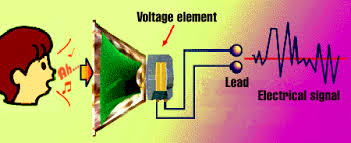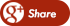﻿ Verilog Examples - Clock Divide by 3Back

## Verilog Examples - Clock Divide by 3A clock Divide by 3 circuit has a clock as an input and it divides the clock input by three. So for example if the frequency of the clock input is 50 MHz, the frequency of the output will be 16.66 MHz. In other words the time period of the outout clock will be thrice the time perioud of the clock input.

The figure shows the example of a clock divider.Problem - Write verilog code that has a clock and a reset as input. It has an output that can be called clk_out. The clk_out is also a clock that has a frequency one third the frequency of the input clock. It has synchronous reset and if there if the reset is 1, the outclock resets to 0. Write test bench to verify it.

Solution -

This is the main code clock.v

 `module clk_div3(clk,reset, clk_out); input clk;input reset;output clk_out; reg [1:0] pos_count, neg_count;wire [1:0] r_nxt; always @(posedge clk)if (reset)pos_count <=0;else if (pos_count ==2) pos_count <= 0;else pos_count<= pos_count +1; always @(negedge clk)if (reset)neg_count <=0;else if (neg_count ==2) neg_count <= 0;else neg_count<= neg_count +1; assign clk_out = ((pos_count == 2) | (neg_count == 2));endmodule`